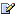CTK Exchange Front Page Movie shortcuts Personal info Awards Reciprocal links Terms of use Privacy Policy Cut The Knot! MSET99 Talk Games & Puzzles Arithmetic/Algebra Geometry Probability Eye Opener Analog Gadgets Inventor's Paradox Did you know?... Proofs Math as Language Things Impossible My Logo Math Poll Other Math sit's Guest book News sit's Recommend this siteCTK Exchange

 Subject: "Sum of 3 mutually prime numbers" Previous Topic | Next Topic
 ConferencesThe CTK ExchangeThis and thatTopic #710Printer-friendly copyEmail this topic to a friend Reading Topic #710
neat_maths
Member since Aug-22-03
May-19-06, 06:46 AM (EST)"Sum of 3 mutually prime numbers"

 It'seems possible to prove that the sum of 3 mutually prime numbers x, y and z cannot contain all the primes contained in each of the pairs (x+y), (y+z) and (x+z).For example x=3, y=4 and z=5 gives (x+y+z)=12 which contains the prime factors in (x+z)=8, (y+z)=9 but not (x+y)=7Can anyone find a counterexample?take care

Subject     Author     Message Date     IDSum of 3 mutually prime numbers neat_maths May-19-06 TOPRE: Sum of 3 mutually prime numbers sfwc May-20-06 1RE: Sum of 3 mutually prime numbers neat_maths May-21-06 2RE: Sum of 3 mutually prime numbers sfwc May-22-06 3RE: Sum of 3 mutually prime numbers erszega May-22-06 4RE: Sum of 3 mutually prime numbers sfwc May-22-06 5RE: Sum of 3 mutually prime numbers neat_maths May-22-06 6RE: Sum of 3 mutually prime numbers sfwc May-23-06 7RE: Sum of 3 mutually prime numbers neat_maths May-24-06 8RE: Sum of 3 mutually prime numbers neat_maths May-27-06 9

 Conferences | Forums | Topics | Previous Topic | Next Topic
sfwc
Member since Jun-19-03
May-20-06, 07:33 AM (EST)1. "RE: Sum of 3 mutually prime numbers"
In response to message #0

 >Can anyone find a counterexample? x = 1, y = -2, z = 3.Thankyousfwc<><neat_maths
Member since Aug-22-03
May-21-06, 11:29 PM (EST)2. "RE: Sum of 3 mutually prime numbers"
In response to message #1

 Good,Now lets exclude -1, 0 and 1Take caresfwc
Member since Jun-19-03
May-22-06, 08:07 AM (EST)3. "RE: Sum of 3 mutually prime numbers"
In response to message #2

 x = -2y = 3z = 5>Good, >Now lets exclude -1, 0 and 1 This seems like rather arbitrary condition. You have said it 'seems possible to prove' this result. Does this mean that you have an idea for how to prove it? If so, please post that idea here. If not, it is unclear why you imposed this condition; if you wish to impose further conditions please give your reasoning.Thankyousfwc<><erszega
Member since Apr-23-05
May-22-06, 02:03 PM (EST)4. "RE: Sum of 3 mutually prime numbers"
In response to message #3

 >x = -2>y = 3>z = 5if we exclude -1, then the choice of x=-2 is not allowed, because a prime number is defined as a positive integer > 1regardssfwc
Member since Jun-19-03
May-22-06, 06:10 PM (EST)5. "RE: Sum of 3 mutually prime numbers"
In response to message #4

 x = 3y = 5z = 22>if we exclude -1, then the choice of x=-2 is not allowed, >because a prime number is defined as a positive integer > 1 I am not sure exactly what you mean by this. Please explain what you understand the condition 'exclude -1, 0 and 1' to mean.Thankyousfwc<><neat_maths
Member since Aug-22-03
May-22-06, 08:24 PM (EST)6. "RE: Sum of 3 mutually prime numbers"
In response to message #5

 Thank you sfwc, x=3, y=5 and z=22 is a good counter example.Back to the drawing board!Can we change the question to the sum of 3 mutually prime integers greater than 1 containing all the primes in each of the 3 numbers?For example x=3, y=5 and z=22 their sum (30) contains 2, 3 and 5 but not 11Take caresfwc
Member since Jun-19-03
May-23-06, 06:45 AM (EST)7. "RE: Sum of 3 mutually prime numbers"
In response to message #6

 >Can we change the question to the sum of 3 mutually prime >integers greater than 1 containing all the primes in each of >the 3 numbers? x = 8, y = 25, z = 27If you want to impose both conditions at once I shall need some time to think about it.Thankyousfwc<><neat_maths
Member since Aug-22-03
May-24-06, 06:22 AM (EST)8. "RE: Sum of 3 mutually prime numbers"
In response to message #7

 Thank you again swfc!You guessed it!It might be possible to prove that no three mutually prime integers greater than 1, say x,y,z can exist where their sum contains all the prime factors in x, y, z, (x+y), (y+z) and (x+z)take careneat_maths
Member since Aug-22-03
May-27-06, 08:02 PM (EST)9. "RE: Sum of 3 mutually prime numbers"
In response to message #7

 Please consider thisIf the sum of three numbers with no common factor contains the prime factors of each of those numbers or of each pair of numbers then it cannot contain any other prime factors. This is because each pair of numbers is a number itself and their sum is twice the sum of the origional numbers. Two pairs can have a common factor but all three pairs cannot have a common factor. Your examples1,2,3 = 1*2*3(3,5,22)*2 == 8,25,27 = 2*2*3*5take care

 Conferences | Forums | Topics | Previous Topic | Next Topic
 Select another forum or conference Lobby The CTK Exchange (Conference)   |--Early math (Public)   |--Middle school (Public)   |--High school (Public)   |--College math (Public)   |--This and that (Public)   |--Guest book (Public) Educational Press (Conference)   |--No Child Left Behind (Public)   |--Math Wars (Public)   |--Mathematics and general education (Public)You may be curious to have a look at the old CTK Exchange archive.